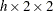ODS Graphics

Statistical procedures use ODS Graphics to create graphs as part of their output. ODS Graphics is described in detail in Chapter 21, Statistical Graphics Using ODS.

Before you create graphs, ODS Graphics must be enabled (for example, with the ODS GRAPHICS ON statement). For more information about enabling and disabling ODS Graphics, see the section Enabling and Disabling ODS Graphics in Chapter 21, Statistical Graphics Using ODS.

The overall appearance of graphs is controlled by ODS styles. Styles and other aspects of using ODS Graphics are discussed in the section A Primer on ODS Statistical Graphics in Chapter 21, Statistical Graphics Using ODS.

When ODS Graphics is enabled, you can request specific plots with the PLOTS= option in the TABLES statement. To produce a weighted frequency plot, you must specify the WTFREQPLOT plot-request in the PLOTS= option. By default, PROC SURVEYFREQ produces all other plots that are associated with the analyses that you request in the TABLES statement. You can suppress default plots and request specific plots by using the PLOTS(ONLY)= option. See the description of the PLOTS= option for more information.

PROC SURVEYFREQ assigns a name to each graph that it creates with ODS Graphics. You can use these names to refer to the graphs. Table 86.8 lists the names of the graphs that PROC SURVEYFREQ generates together with their descriptions, their PLOTS= options (plot-requests), and the TABLES statement options that are required to produce the graphs.

Table 86.8 ODS Graphs Produced by PROC SURVEYFREQ

ODS Graph Name

Description

PLOTS= Option

TABLES Statement Option

WtFreqPlot

Weighted frequency plot

Any table request

ORPlot

Odds ratio plot

OR (table)

RelRiskPlot

Relative risk plot

OR (table)

RiskDiffPlot

Risk difference plot

RISK (table)